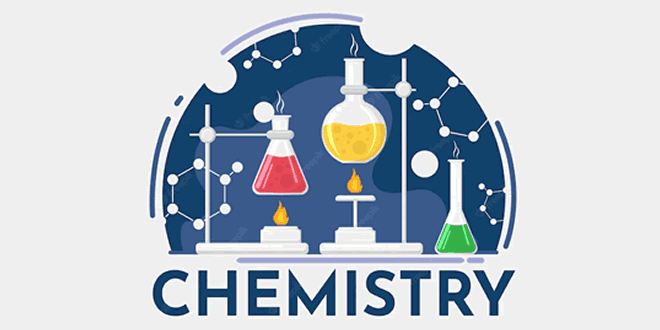Tuesday , August 9 2022# 12th Class Chemistry Pre-Mid Term Examination 2022-23

 School Name: Himalaya Public School, Sector 13, Rohini, Delhi 110085 India Class: 12th Standard (CBSE) Subject: Chemistry Time Duration: 01 Hours Maximum Marks: 30 Date: 29 / 06 / 2022

### General Instructions: 12th Pre-Mid Term Chemistry Examination

• Questions 1 to 5 carry 1 marks each
• Questions 6 to 9 carry 2 marks each
• Questions 10 to 13 carry 3 marks each
• Question 14 to carry 5 marks

#### Question 01:

Define reverse osmosis?

#### Question 02:

What is the importance of Henry’s Law Constant?

#### Question 03:

What role does molecular interaction play in a solution of alcohol and water?

#### Question 04:

If the molality of dil solution is doubled, the value of molal depression constant Kf will be _______ .

#### Question 05:

Write the structure of 4-tertbutyl-3-iodoheptane.

#### Question 06:

If N2 gas is bubbled through water at 293K, how many mill moles of N2 gas would be dissolved in one litre of water? Assume that N2 exerts a partial pressure of 0.987 bar. Given that Henry’s Law Constant for N2 at 293k is 76.48kbar.

#### Question 07:

Write the lupac name of the following compounds and classify them as well

i. CH3 = C(Cl)CH2Cj(CH3)2
ii. o-Br-C6H4CH(CH3)Ch2CH3

#### Question 08:

Derive a relationship between relative lowering of vapour Pressure and molar mass of solute.

#### Question 09:

Calculate the boiling point of 1 molar solution of solute (molar mass 74.5 g / mol)

#### Question 10:

Complete the following reactions:

Acetone
1.
CH3CH2CH2Cl→
2.
CH2CH2OH + HBr (Heat)→
3.
CH(CH3)2 + Br2→

#### Question 11:

An aqueous solution of 3.12g of BaCl2 in 250g water is found to boil at 100.832°C. Calculate the degree of dissolution of BaCl2 (kb=0.52K/m)

#### Question 12: 12th Pre-Mid Term Chemistry Examination

1. How does mole fraction of HCL gas in its solution in cyclo hexane varies with partial pressure of HCL(g)?
2. Show with the help of graph?
3. How can we calculate the value of KH with the help of graph?

#### OR

1. How will you differentiate between Sandmayer and Gattermann reaction. (any two difference including reaction)
2. The reaction of C6H5-CH = CH-CH3 with HBr produces _________ .

#### Question 13:

Define the following:

1. Finkelstein reaction
2. Balzscheimann reaction
3. Melting point of isomeric haloarene

#### Question 14:

The properties of the solutions which depends only on the number of solute particles but not on the nature of the solute are called colligative properties. Relative lowering in vapour pressure is also an example of colligative properties. For an experiment, sugar solution is prepared for which lowering in vapour was found to be 0.061 mm of Hg. (vapour pressure of water at 200 C is 17.5 mm of Hg.

The following questions are multiple choice questions. Choose the most appropriate answer

1. Relative lowering of vapour pressure for the given solution is
1. 0.00348
2. 0.061
3. 0.122
4. 1.75
2. The vapour pressure (mm of Hg) of solution will be
1. 17.5
2. 0.61
3. 17.439
4. 0.00348
3. Mole fraction of sugar in the solution is
1. 0.00348
2. 0.9965
3. 0.061
4. 1.75
4. If weight of sugar taken is 5 g in 108 g of water then molar mass of sugar will be
1. 358
2. 120
3. 240
4. 400
5. The vapour pressure (mm of Hg) of water at 293 K when 25 g of glucose is dissolved in 450 g of water is
1. 17.2
2. 17.4
3. 17.120
4. 17.02

## 11th Class English Term 2 Question Paper 2021-22

School Name: Himalaya Public School, Sector 13, Rohini, Delhi 110085 India Class: 11th Standard (CBSE) Subject: English Time …